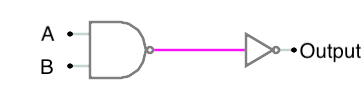## Lesson goal: Prolog and digital electronics

Previous: Solving a right triangle | Home | Next: Simulating digital circuits (2)

Basic digital electronics is based around some fundamental gates: the AND, OR, XOR and NOT gates (and/or NAND or NOR gates). These have the "truth tables" and symbols as follows.As you can see, an inveter takes in a binary input on its A input, and flips it on the B output (a 1 becomes a 0 and a 0 becomes a 1). The other gates have two inputs, A and B, with their output being C. The AND gate for example, only outputs a 1 only if both of its inputs, A and B, are 1 as well. XOR only outputs a 1 if either of its inputs (A and B) are 1, but not both of them. These "basic" gates can be represented in Prolog farily easily as you can see in the code below. (In the code, the output of a gate is always in the last position. The inputs are in the first (inverter) or first and second (all others) positions.)

More complicated circuts can be made from these basics units. Let's build some and have Prolog "simulate" what the truth table for it will be.

First, we'll put in the rules for just an NAND gate and an inverter as shown here:Then, we'll run a goal that simulates this circuit: